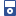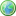Accessibility options:

# Geometry resources

Show me all resources applicable to

### iPOD Video (6)Equations of a Straight Line Part 1
IPOD VIDEO: In this unit we find the equation of a straight line, when we are given some information about the line. The information could be the value of its gradient, together with the co-ordinates of a point on the line. Alternatively, the information might be the co-ordinates of two different points on the line. There are several different ways of expressing the final equation, and some are more general than others. This resource is released under a Creative Commons license Attribution-Non-Commercial-No Derivative Works and the copyright is held by Skillbank Solutions Ltd.Equations of a Straight Line Part 2
IPOD VIDEO: In this unit we find the equation of a straight line, when we are given some information about the line. The information could be the value of its gradient, together with the co-ordinates of a point on the line. Alternatively, the information might be the co-ordinates of two different points on the line. There are several different ways of expressing the final equation, and some are more general than others. This resource is released under a Creative Commons license Attribution-Non-Commercial-No Derivative Works and the copyright is held by Skillbank Solutions Ltd.Equations of a Straight Line Part 3
IPOD VIDEO: In this unit we find the equation of a straight line, when we are given some information about the line. The information could be the value of its gradient, together with the co-ordinates of a point on the line. Alternatively, the information might be the co-ordinates of two different points on the line. There are several different ways of expressing the final equation, and some are more general than others. This resource is released under a Creative Commons license Attribution-Non-Commercial-No Derivative Works and the copyright is held by Skillbank Solutions Ltd.Equations of a Straight Line Part 4
IPOD VIDEO: In this unit we find the equation of a straight line, when we are given some information about the line. The information could be the value of its gradient, together with the co-ordinates of a point on the line. Alternatively, the information might be the co-ordinates of two different points on the line. There are several different ways of expressing the final equation, and some are more general than others. This resource is released under a Creative Commons license Attribution-Non-Commercial-No Derivative Works and the copyright is held by Skillbank Solutions Ltd.Equations of a Straight Line Part 5
IPOD VIDEO: In this unit we find the equation of a straight line, when we are given some information about the line. The information could be the value of its gradient, together with the co-ordinates of a point on the line. Alternatively, the information might be the co-ordinates of two different points on the line. There are several different ways of expressing the final equation, and some are more general than others. This resource is released under a Creative Commons license Attribution-Non-Commercial-No Derivative Works and the copyright is held by Skillbank Solutions Ltd.Equations of a Straight Line Part 5
IPOD VIDEO: In this unit we find the equation of a straight line, when we are given some information about the line. The information could be the value of its gradient, together with the co-ordinates of a point on the line. Alternatively, the information might be the co-ordinates of two different points on the line. There are several different ways of expressing the final equation, and some are more general than others. This resource is released under a Creative Commons license Attribution-Non-Commercial-No Derivative Works and the copyright is held by Skillbank Solutions Ltd.

### Teach Yourself (3)Equations of a straight line
In this unit we find the equation of a straight line, when we are given some information about the line. The information could be the value of its gradient, together with the co-ordinates of a point on the line. Alternatively, the information might be the co-ordinates of two different points on the line. There are several different ways of expressing the final equation, and some are more general than others.Properties of straight line segments
In this unit we use a system of co-ordinates to find various properties of the straight line between two points. We find the distance between the two points and the mid-point of the line joining the two points.The gradient of a straight line segment
In this unit we find the gradient of a straight line segment, and the relationships between the gradients of parallel lines and of perpendicular lines.

### Test Yourself (1)Maths EG
Computer-aided assessment of maths, stats and numeracy from GCSE to undergraduate level 2. These resources have been made available under a Creative Common licence by Martin Greenhow and Abdulrahman Kamavi, Brunel University.

Website design by Pink Mayhem, Leicester